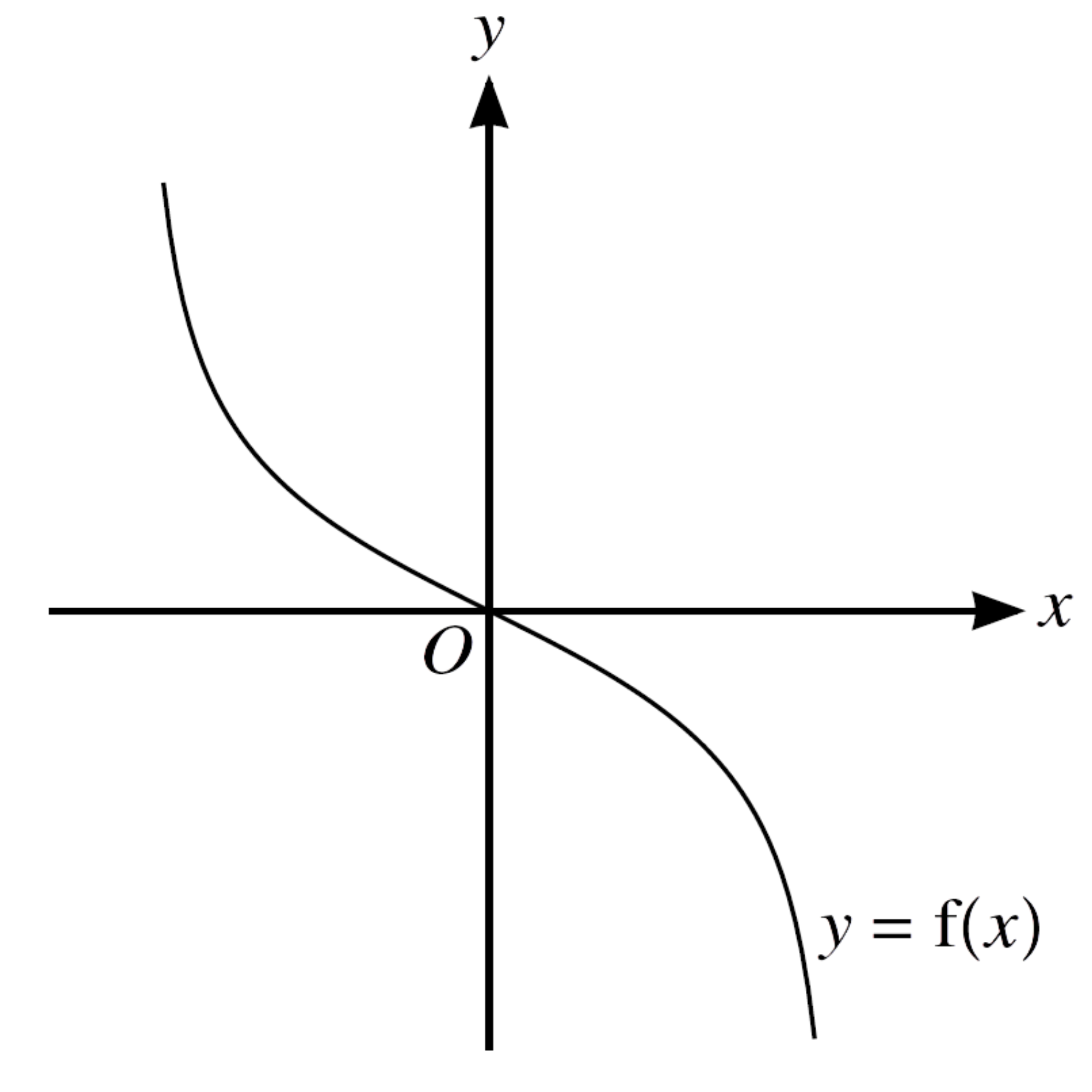Home » 9709 » Paper 13 Oct Nov 2021 Pure Math I – 9709/13/O/N/21

Check out my complete solution here:
» Full Solutions «
Like and subscribe too! =)

1.     The graph of $y = \mathrm{f}(x)$ is transformed to the graph of $y = 3 \ − \ \mathrm{f}(x)$.

Describe fully, in the correct order, the two transformations that have been combined.
$$\tag*{}$$

2.     (a)     Find the first three terms, in ascending powers of $x$, in the expansion of ${(1 \ + \ ax)}^{6}$.
$$\tag*{}$$
(b)     Given that the coefficient of $x^2$ in the expansion of $(1 \ − \ 3x){(1 \ + \ ax)}^{6}$ is −3, find the possible values of the constant $a$.
$$\tag*{}$$

3.     (a)     Express $5{y}^{2} \ − \ 30y \ + \ 50$ in the form $5{(y \ + \ a)}^{2} \ + \ b$, where $a$ and $b$ are constants.
$$\tag*{}$$
(b)     The function $\mathrm{f}$ is defined by $\mathrm{f}(x) = x^5 \ – \ 10{x}^{3} \ + \ 50x$ for $x \in \mathbb{R}$.

Determine whether $\mathrm{f}$ is an increasing function, a decreasing function or neither.
$$\tag*{}$$

4.     The first term of an arithmetic progression is 84 and the common difference is −3.

(a)    Find the smallest value of $n$ for which the nth term is negative.
$$\tag*{}$$
It is given that the sum of the first $2k$ terms of this progression is equal to the sum of the first $k$ terms.

(b)     Find the value of $k$.
$$\tag*{}$$

5.In the diagram, $X$ and $Y$ are points on the line $AB$ such that $BX = 9$ cm and $AY = 11$ cm. Arc $BC$ is part of a circle with centre $X$ and radius 9 cm, where $CX$ is perpendicular to $AB$. Arc $AC$ is part of a circle with centre $Y$ and radius 11 cm.

(a)     Show that angle $XYC = 0.9582$ radians, correct to 4 significant figures.
$$\tag*{}$$
(b)     Find the perimeter of ABC.
$$\tag*{}$$

6.The diagram shows the graph of $y = \mathrm{f}(x)$.

(a)     On this diagram sketch the graph of $y = {\mathrm{f}}^{-1}(x)$.
$$\tag*{}$$
It is now given that $\displaystyle \mathrm{f}(x) = – \frac{x}{\sqrt{4 \ – \ {x}^{2}}}$ where $-2 \lt x \lt 2$.

(b)     Find an expression for ${\mathrm{f}}^{-1}(x)$.
$$\tag*{}$$
The function $\mathrm{g}$ is defined by $\mathrm{g}(x) = 2x$ for $−a \lt x \lt a$, where $a$ is a constant.

(c)     State the maximum possible value of a for which $\mathrm{fg}$ can be formed.
$$\tag*{}$$
(d)     Assuming that $\mathrm{fg}$ can be formed, find and simplify an expression for $\mathrm{fg}(x)$.
$$\tag*{}$$

7.     (a)     Show that the equation $\displaystyle \frac{\tan x + \cos x}{\tan x – \cos x} = k$, where $k$ is a constant, can be expressed as
$$(k \ + \ 1) {\sin}^{2}x + (k \ – \ 1) \sin x \ – \ (k \ + \ 1) = 0.$$
$$\tag*{}$$
(b)     Hence solve the equation $\displaystyle \frac{\tan x + \cos x}{\tan x – \cos x} = 4$ for $0 \le x \le {360}^{\circ}$.
$$\tag*{}$$

8.The diagram shows the curves with equations $y = {x}^{-\frac{1}{2}}$ and $y = \frac{5}{2} \ – \ {x}^{\frac{1}{2}}$. The curves intersect at the points $A(\frac{1}{4}, 2)$ and $B(4, \frac{1}{2})$.

(a)     Find the area of the region between the two curves.
$$\tag*{}$$
(b)     The normal to the curve $y = {x}^{−\frac{1}{2}}$ at the point $(1, 1)$ intersects the $y$-axis at the point $(0, p)$. Find the value of $p$.
$$\tag*{}$$

9.     The line $y = 2x \ + \ 5$ intersects the circle with equation $x^2 \ + \ y^2 = 20$ at $A$ and $B$.

(a)     Find the coordinates of $A$ and $B$ in surd form and hence find the exact length of the chord $AB$.
$$\tag*{}$$
A straight line through the point $(10, 0)$ with gradient $m$ is a tangent to the circle.

(b)     Find the two possible values of $m$.
$$\tag*{}$$

10.     A curve has equation $y = \mathrm{f}(x)$ and it is given that
$${\mathrm{f}}^{‘}(x) = {(\frac{1}{2}x \ + \ k)}^{-2} \ – \ {(1 \ + \ k)}^{-2},$$
where $k$ is a constant. The curve has a minimum point at $x = 2$.

(a)     Find ${\mathrm{f}}^{”}(x)$ in terms of $k$ and $x$, and hence find the set of possible values of $k$.
$$\tag*{}$$
It is now given that $k = −3$ and the minimum point is at $(2, 3\frac{1}{2})$.

(b)     Find $\mathrm{f}(x)$.
$$\tag*{}$$
(c)     Find the coordinates of the other stationary point and determine its nature.
$$\tag*{}$$